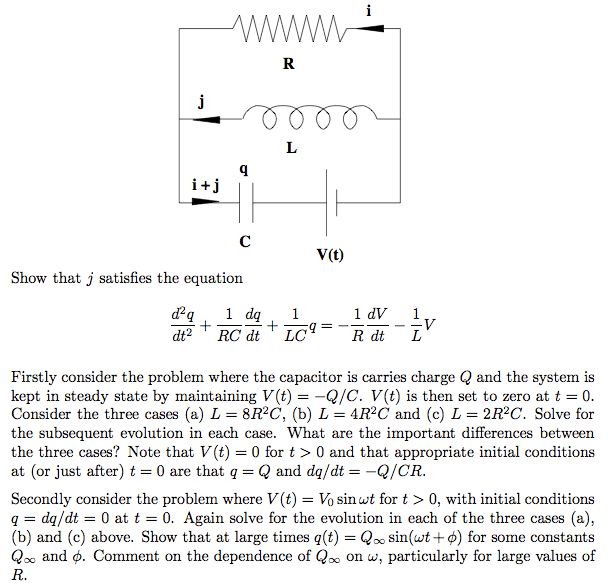# RLC Circuit Homework - Solving 3rd Part with 2nd Order DE

• bigevil

## Homework Statement## The Attempt at a Solution

Ok, first part, no problem, second part (steady state), solved in another thread. Both are pretty tedious, but doable.

However, I am quite stumped by the third part of this problem (V = V_o sin wt) .

The second part of the problem requires me to use a 2nd order differential equation (y'' + ay' + b = 0), and the solutions of the auxiliary equation can be saved for the third part.

For the third part, I choose a particular integral y = A sin wt + B cos wt. The last part arises essentially because the GS of the third part is y = exponent + exponent + A sin wt + B cos wt, and the first two terms become 0 when t is large. Then I can reexpress A sin wt + B cos wt into the form required.

I have just tried to go about the third part the standard way (differentiating and substituting the particular integral to find A and B, and then substituting again to find the constants in the complementary function), but it is extremely tedious (one page working just to find and simplify A or B) and I hope there is a shorter solution.

There is a well-known trick. V_0 sin wt is the imaginary part of V_0 exp(iwt).
i.e. V_0 sin wt = Im( V_0 exp(iwt) )

Also, since the coefficients of the differential equation are real, real and imaginary part of the left and the right hand side of the equation behaves independently. Hence, the solution you are looking for is the imaginary part of the solution for V_0 exp(iwt).

Try to solve for V_0 exp(iwt). It simplifies the problem considerably.